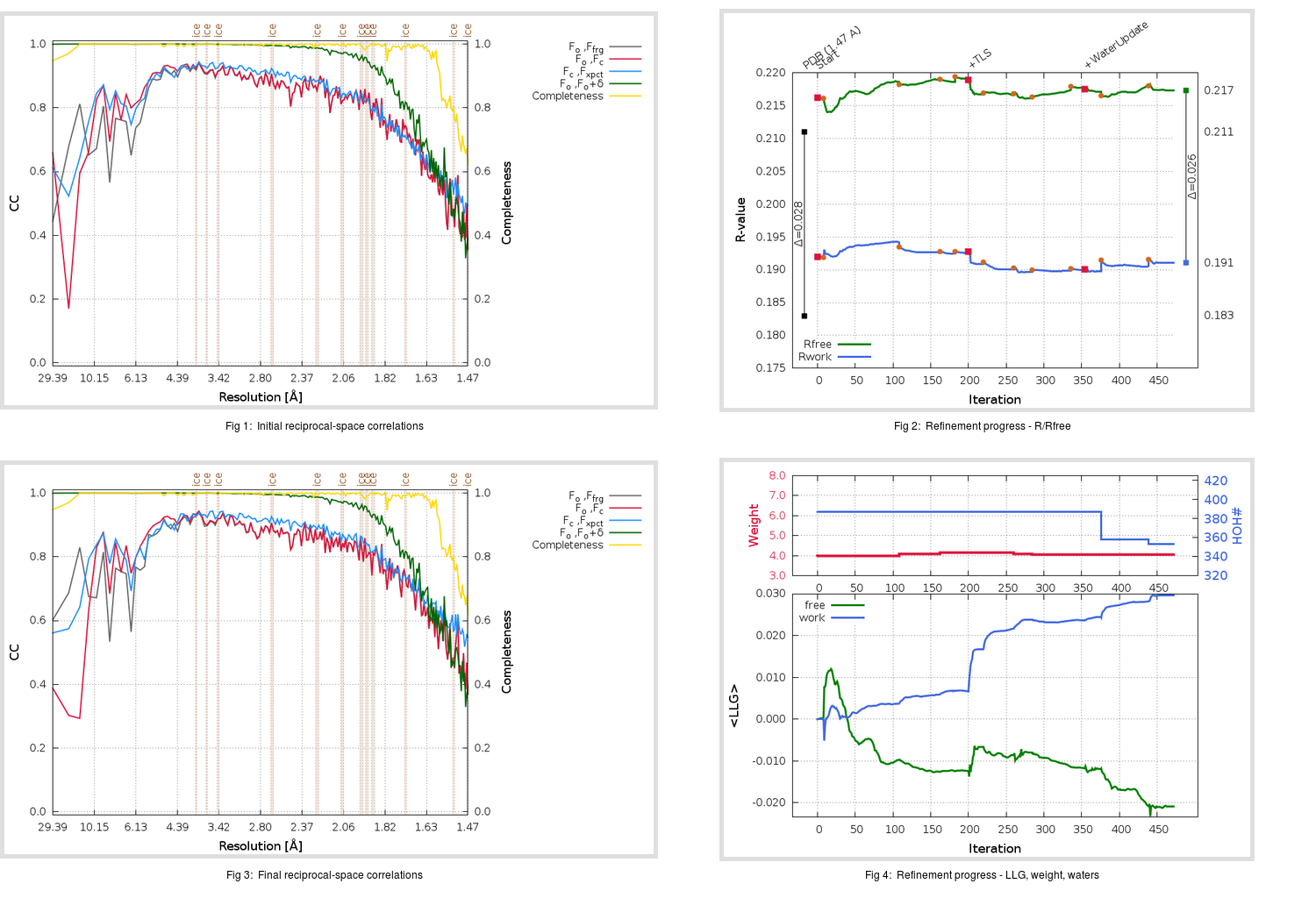Content:

```    Diffraction limits & principal axes of ellipsoid fitted to diffraction cut-off surface:
1.608         0.9525   0.0000  -0.3044       0.979 a* - 0.206 c*
1.564         0.0000   1.0000   0.0000       b*
1.475         0.3044   0.0000   0.9525       0.670 a* + 0.742 c*
```

## Deposited

` `
 Date deposited Date data collection Resolution R, Rfree 20200315 20200227 1.47 0.1810 0.2110

Molprobity (CCP4 7.0 version) summary:

```Ramachandran outliers =   0.33 %
favored =  97.68 %
Rotamer outliers      =   1.07 %
C-beta deviations     =     1
Clashscore            =   6.76
RMS(bonds)            =   0.0138
RMS(angles)           =   1.83
MolProbity score      =   1.46
Resolution            =   1.47
R-work                =   0.1810
R-free                =   0.2110
```

```Number of waters      =   387

<B> (all atoms) =   23.51 ( sd =   10.99 ) for       2922 non-hydrogen atoms
<B>   (protein) =   21.28 ( sd =    9.24 ) for       2508 non-hydrogen atoms
<B>     (water) =   35.85 ( sd =   10.98 ) for        387 non-hydrogen atoms
<B>    (others) =   36.50 ( sd =   18.12 ) for         27 non-hydrogen atoms

B min/max       (all non-hydrogen atoms) =   11.18 /   80.44
B min/max   (protein non-hydrogen atoms) =   11.18 /   80.44
B min/max     (water non-hydrogen atoms) =   12.94 /   71.55
B min/max     (other non-hydrogen atoms) =   20.99 /   68.83
```

## BUSTER (re-)refinement

` `

Molprobity (CCP4 7.0 version) summary:

```Ramachandran outliers =   0.33 %
favored =  98.34 %
Rotamer outliers      =   1.07 %
C-beta deviations     =     0
Clashscore            =   3.38
RMS(bonds)            =   0.0117
RMS(angles)           =   1.57
MolProbity score      =   1.15
Resolution            =   1.47
R-work                =   0.1911
R-free                =   0.2173
```

```Number of waters      =   353

<B> (all atoms) =   24.37 ( sd =    9.93 ) for       2888 non-hydrogen atoms
<B>   (protein) =   22.20 ( sd =    7.65 ) for       2508 non-hydrogen atoms
<B>     (water) =   36.98 ( sd =   10.67 ) for        353 non-hydrogen atoms
<B>    (others) =   47.19 ( sd =   25.36 ) for         27 non-hydrogen atoms

B min/max       (all non-hydrogen atoms) =    5.72 /   87.85
B min/max   (protein non-hydrogen atoms) =    5.72 /   60.96
B min/max     (water non-hydrogen atoms) =   15.07 /   83.67
B min/max     (other non-hydrogen atoms) =   24.84 /   87.85
```

Refinement progression:Results:

` `
 File Remark 5RED_aB_refine.01_03_refine.pdb.gz exact refinement commands are in header 5RED_aB_refine.01_03_refine.mtz.gz including original deposited data and several re-refinement map coefficients 5RED_aB_refine.01_03_BUSTER_model.cif.gz including any non-standard compound restraints 5RED_aB_refine.01_03_BUSTER_refln.cif.gz